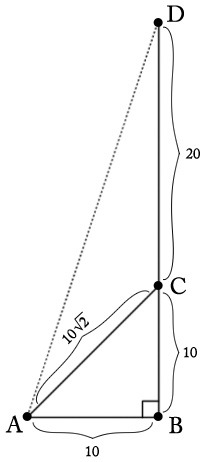# Points from Directions

Alignments to Content Standards: 8.G.B.7 G-SRT.B.5

Point $B$ is due east of point $A$. Point $C$ is due north of point $B$. The distance between points $A$ and $C$ is $10\sqrt 2$ meters, and $\angle BAC = 45^{\circ}$. Point $D$ is 20 meters due north of point $C$. The distance $|AD|$ is between which two integers?

## IM Commentary

This task provides a slightly more involved use of similarity, requiring students to translate the given directions into an accurate picture, and persevere in solving a multi-step problem: They must calculate segment lengths, requiring the use of the Pythagorean theorem, and either know or derive trigonometric properties of isosceles right triangles (with the distinction between these two cases dictating its level of appropriateness for an 8th grade or high school task, and possibly the extent to which the task is appropriate for assessment).

This task was adapted from problem #12 on the 2012 American Mathematics Competition (AMC) 10B Test. For the 2012 AMC 10B, which was taken by 35,086 students, the multiple choice answers for the problem had the following distribution:

 Choice Answer Percentage of Answers (A) 30 and 31 8.76 (B)* 31 and 32 55.82 (C) 32 and 33 5.29 (D) 33 and 34 5.24 (E) 34 and 35 3.32 Omit -- 21.55

Of the 35,086 students: 17,169, or 49%, were in 10th grade; 9,928 or 28%, were in 9th grade; and the remainder were below 9th grade.

## Solutions

Solution: 1 Pythagorean Theorem

The information from the problem statement is shown in the picture below:We know that $m(\angle BAC) = 45$ and $m(\angle ABC) = 90$. Since the sum of angles in a triangle is $180^\circ$ we can conclude that $m(\angle BCA)= 45$. This means that $\triangle ABC$ is isosceles with $|AB| = |BC|$. To find these lengths, we can use the Pythagorean theorem: $$|AB|^2 + |BC|^2 = |AC|^2.$$ Substituting $|AB| = |BC|$ and $|AC| = 10\sqrt{2}$ this equation becomes $$2|BC|^2 = (10\sqrt{2})^2 = 200.$$ Solving this we find $|BC| = 10$ and so $|AC| = 10$ as well.

We have $|BD| = |BC| + |CD| = 10 + 20 = 30$ and we can apply the Pythagorean theorem a second time, to the right triangle $ABD$, to calculate the length of its hypotenuse $|AD|$:

\begin{align} |AD|^2 &= |AB|^2 + |BD|^2 \\ &= 10^2 + 30^2 \\ & = 1000 \end{align} <\div>

We have that $31^2 = 961$ and $32^2 = 1024$ so this means that $31 \lt |AD| \lt 32$.

Solution: 2 Similar Triangles

The information from the problem statement is shown in the picture below:We are given that $D$ is due north of $C$ and $A$ is due east of $B$ so angle $ABD$ is a right angle. We are also given that angle $BAC$ is a $45^\circ$ angle. Since the sum of the angles in $\triangle ABC$ is $180^\circ$ this means that $m(\angle BCA) = 45$. In particular $\triangle ABC$ is a right isosceles triangle. One right isosceles triangle has leg lengths of $1$ and hypotenuse $\sqrt{2}$. All right isosceles triangles are similar and since the hypotenuse of $\triangle ABC$ measures $10 \times \sqrt{2}$ this means that the scale factor between the $(1,1,\sqrt{2})$ right isosceles triangle and $\triangle ABC$ must be $10$. So $|AB| = |BC| = 10 \times 1 = 10$. We have $$|BD| = |BC|+|CD|= 10+20=30.$$ We know $\triangle ABD$ is a right triangle so the Pythagorean Theorem gives

\begin{align} |AD| & \sqrt{|AB|^2 + |BD|^2} \\ &= \sqrt{10^2 + 30^2} \\ &= \sqrt{1000}. \end{align} <\div>

We have $31^2=961$ and $32^2=1024$ so $31 \lt |AD| \lt 32$.